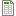# BA 215 Project Week 7

For these project assignments throughout the course you will need to reference the data in the ROI Excel spreadheet. Download it here.

Using the ROI data set:
For each of the two majors:

1. Draw the scatter diagram of Y = ‘Annual % ROI’ against X = ‘Cost’.
2. Obtain b0 and b1 of the regression equation defined as y ̂ = b0 + b1X and the coefficient of determination (r2) from the Excel regression output.
3. Draw the fitted regression line on the scatter diagram.
4. Calculate the estimated ‘Annual % ROI’ when the ‘Cost’ (X) is \$160,000.
5. Test the hypothesis:
• H0: β1 = 0
• Ha: β1 ≠ 0
6. Write a paragraph or more on any observations you make about the regression estimates, coefficient of determination, the plots, and the results of your hypothesis tests.
Attachments:spreadsheet_week_7.xlsx
Field of study:
Date Due:
Saturday, October 20, 2018